# 空心线圈传感器的变阻尼标定方法研究 Research on Variable Damping Calibration Method of Air-Core Coil Sensor

DOI: 10.12677/JSTA.2020.84013, PDF, HTML, XML, 下载: 183  浏览: 420

Abstract: The inductive air-core coil sensor is an important time-varying magnetic field measurement tool. Due to the inherent inductance and distributed capacitance of the coil, the output voltage of the coil cannot accurately follow the sudden induced voltage. This phenomenon is called the transition process of the coil. The existing correction methods can only be completed by the laboratory, and it is difficult to calibrate the parameter disturbance caused by the field environment. This paper takes the air-core coil sensor used in transient electromagnetic detection as an example, and proposes a scheme to eliminate the transition process by using the transfer function. By analyzing the equivalent circuit of the receiver coil, a variable damping coil calibration method suitable for solving the parameters of the receiver coil in the field environment is proposed. The device has a simple form and high reliability, which meets the needs of field calibration in the construction environment.

1. 引言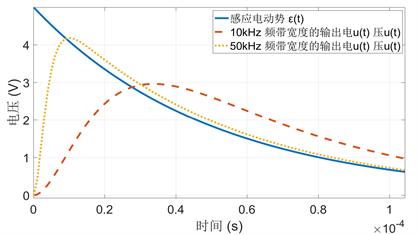Figure 1. The effect of the transition process of the receiving coil on the signal

2. 空心线圈的过渡过程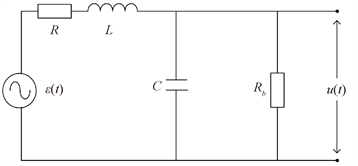Figure 2. Equivalent circuit of receiving coil distributed parameter

$H\left(s\right)=\frac{u\left(s\right)}{\epsilon \left(s\right)}=\frac{1}{{s}^{2}LC+s\left(\frac{L}{{R}_{b}}+RC\right)+\frac{R+{R}_{b}}{{R}_{b}}}$ (1)

$\frac{\epsilon \left(t\right)}{LC}=\frac{{\text{d}}^{2}u\left(t\right)}{\text{d}{t}^{2}}+2\delta \frac{\text{d}u\left(t\right)}{\text{d}t}+{\omega }_{p}^{2}u\left(t\right)$ (2)

$\xi =\frac{{\delta }_{1}}{{\omega }_{p}}=\frac{{R}_{b}RC+L}{2\sqrt{LC{R}_{b}\left(R+{R}_{b}\right)}}$ (3)

${R}_{0}=\frac{L}{RC+2\sqrt{LC}}$ (4)

3. 变阻尼标定法

$\iint v\left(t\right)\text{d}{t}^{2}=\frac{{\tau }_{l}{\tau }_{c}}{\alpha }u\left(t\right)+\left({\tau }_{l}+{\tau }_{c}\right)\int u\left(t\right)\text{d}t+\left(1+\alpha \right)\iint u\left(t\right)\text{d}{t}^{2}$ (5)

0.8倍关系选取三个阻尼电阻值，获得三个参数方程。

$\left\{\begin{array}{l}V\left(t\right)={m}_{1}×{u}_{1}\left(t\right)+{m}_{2}×\int {u}_{1}\left(t\right)\text{d}t+\left(1+\alpha \right)×\iint {u}_{1}\left(t\right)\text{d}{t}^{2}\\ V\left(t\right)={m}_{1}×{u}_{2}\left(t\right)+{m}_{2}×\int {u}_{2}\left(t\right)\text{d}t+\left(1+\alpha \right)×\iint {u}_{2}\left(t\right)\text{d}{t}^{2}\\ V\left(t\right)={m}_{1}×{u}_{3}\left(t\right)+{m}_{2}×\int {u}_{3}\left(t\right)\text{d}t+\left(1+\alpha \right)×\iint {u}_{3}\left(t\right)\text{d}{t}^{2}\end{array}$ (6)

${u}_{2}\left(t\right)$${u}_{3}\left(t\right)$ 可以通过A/D采样直接得到。求解方程组(6)便可以确定参数 ${m}_{1}$${m}_{2}$，进而获取线圈的传递函数 $H\left(s\right)$

$\eta =\mathrm{max}\left\{\begin{array}{l}|\frac{L-{L}_{ct}}{L}|\ast 1000\\ |\frac{C-{C}_{ct}}{C}|\ast 1000\end{array}\begin{array}{c}‰\\ \begin{array}{l}\\ ‰\end{array}\end{array}$ (7)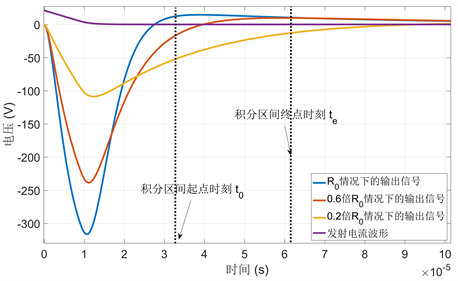Figure 3. The measured voltage curve of the variable damping calibration method

4. 讨论

4.1. 采样率对结果的影响

4.2. 阻尼电阻Rb对结果的影响

${R}_{b}$ 按临界值的不同倍数关系选取三个阻尼电阻值，在相同的激励源作用下，得到三组响应信号 ${u}_{1}\left(t\right)$${u}_{2}\left(t\right)$${u}_{3}\left(t\right)$ 组成方程组，考察阻尼电阻的选取对参数求解精度的影响。Table 1. The influence of sampling rate on parameter solution error rateTable 2. The relationship between parameter error rate and tolerance of damping resistance

4.3. 积分区间对结果的影响Table 3. The influence of the integration starting point on the error rate of parameter solution

5. 实验结果(a)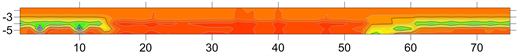(b)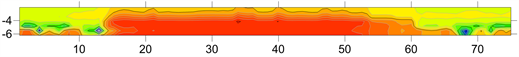(c)

Figure 4. Comparison of apparent resistivity imaging results before and after the transition process, (a) the schematic diagram of the air-raid shelter distribution; (b) before the transition process; (c) after the transition process

6. 小结

  Smith, R.S. and Balch, S.J. (2000) Robust Estimation of the Band-Limited Inductive-Limit Response from Im-pulse-Response TEM Measurements Taken during the Transmitter Switch-Off and the Transmitter Off-Time. Theory and an Example from Voisey’s Bay, Labrador, Canada. Geophysics, 65, 476-481. https://doi.org/10.1190/1.1444741  Wang, Z., Lin, J., Yu, S.B., Ji, Y.J. and Zhou, G.H. (2006) ATTEM: An Instrument System Using Transient Electromagnetic Pulse for Subsurface Imaging. IEEE Instrumentation and Meas-urement Technology Conference, Sorrento, 2231-2235.  Spies, B.R. (1989) Depth of Investigation in Electromag-netic Sounding Methods. Geophysics, 54, 872. https://doi.org/10.1190/1.1442716  谢伟伦, 黄志威, 刘小沛. 杂散电容对CVT谐波测量影响的处理方法研究[J]. 电力电容器与无功补偿, 2020, 41(2): 59-63.  Yu, C.G. and Fu, Z.H. (2014) Transient Process and Op-timal Design of Receiver Coil for Small-Loop Transient Electromagnetics. Geophysical Prospecting, 62, 377-384. https://doi.org/10.1111/1365-2478.12093  Lin, J. (2014) Research and Development on the Air-Core Coil Sensor for Mine Transient Electromagnetic Exploration. Chinese Journal of Geophysics, 59, 721-730.  Chu, X.Y. and Zhang, S. (2012) An Optimal Transfer Characteristic of an Air Cored Transient Electromagnetic Sensor. 2012 Inter-national Conference on Industrial Control and Electronics Engineering, Xi’an, 23-25 August 2012, 482-485. https://doi.org/10.1109/ICICEE.2012.134  Hemmati, E. and Shahrtash, S.M. (2013) Digital Compensation of Rogowski Coil’s Output Voltage. IEEE Transactions on Instrumentation and Measurement, 62, 71-82. https://doi.org/10.1109/TIM.2012.2212502  万云霞, 王言章, 程德福. 感应式磁传感器标定磁场处理方法研究[J]. 传感技术学报, 2013, 26(4): 520-524.  Gurleyuk, S.S., Taskin, H. and Sarac, Z. (2012) Measurement of the Parameters and the Resonance Frequency in Semiconductor Controlled Tesla Transformer. International Journal of Electrical Power and Energy Systems, 43, 6-10. https://doi.org/10.1016/j.ijepes.2012.05.026  Kozhevnikov, N.O. (2012) Testing TEM Systems Using a Large Horizontal Loop Conductor. Russian Geology and Geophysics, 53, 1243-1251. https://doi.org/10.1016/j.rgg.2012.09.010  Persova, M.G., Soloveichik, Y.G., Trigubovich, G.M., et al. (2014) Transient Electromagnetic Modelling of an Isolated Wire Loop over a Conductive Medium. Geophysical Prospecting, 62, 1193-1201. https://doi.org/10.1111/1365-2478.12122  Davis, A. and Macnas, J. (2008) Quantifying AEM System Char-acteristics Using a Ground Loop. Geophysics, 4, F179-F188. https://doi.org/10.1190/1.2943189  Wang, H.M., Liu, F., Zhang, H.L. and Zheng, S.X. (2005) Analysis of the Thermal Expansion Effect on Measurement Precision of Rogowski Coils. International Conference on Power Electronics and Drives Systems, Kuala Lumpur, 1658-1661.  林君, 王琳, 王晓光, 等. 矿井瞬变电磁探测中空芯线圈传感器的研制[J]. 地球物理学报, 2016, 59(2): 721-730.